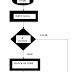If statement in C is a decision-making statement (in real life, we need to take some decision, these decisions decide what should we do next, the same condition for programming which determines what block of code will execute). When the condition in if statement is true, then the block of code will execute and condition in if statement is false, then the block of code will not execute.

Syntax
if (condition) {
//Block of code

### Flow Chart of if Statement in C

If curly braces ({ ) is not provided after if statement, then only the first statement will execute, and the rest of the statement will not execute.

### Program 1: Write a program to find out the largest number from the given numbers are 14 & 13 using if statement in C.

Here, we tried to explain how to find out the largest number step by step:
Algorithm
Step1:  Start the program.
Step2:  Enter input data a =14, b=13.
Step3: Write if statement with the condition (a>b).
Step4:  When the condition (a>b) is true, then the block of code will execute. When the condition (a>b) is false, then go to Step5.
Step5: Print the output.
Step6: Finish the program.

### Flow Chart of the Largest Number Program

Program
#include <stdio.h>
int main()
{
int a =14, b 13;
if (> b)
{
printf ("largest number is=%d\n", a);
}
return 0;
}

Output
largest number is = 14

### Program 2: Write a program to count the number of boys whose weight is less than 50 kg and height is greater than 170 cm using if statement in C.

Steps mentioned below helps you to understand the program,
Algorithm
Step1: Start the program.
Step2: Input data number_of_boys, i, count=0, weight, and height.
Step3: Write condition in if statement (weight should be less than 50 kg and height should be greater than 170 cm).
Step4: When if the condition is true, then the number of count of boys will increase by one. When the condition is false, then go to Step5.
Step5: Print the output.
Step6: Stop the program.

### Flow Chart

Program
#include <stdio.h>
int main()
{
int number_of_boys, i, count = 0;
float weightheight;
printf ("Enter the number of boys\n");
scanf ("%d", &number_of_boys);
printf ("Entered number_of_boys are %d\n", number_of_boys);
printf ("Enter the number of weight and height");
for (=1;i<=number_of_boys; i++)
{
scanf ("%f%f", &weight, &height);
if (weight < 50 && height >170)
{
count ++;
}
}
printf ("The number of boys with weight less than 50kg and height greater than 170cm is %d\n"count);
return 0;
}

Output
Enter the number of boys
5
Entered number_of_boys are 5
Enter the number of weight and height 45 172
46 178
51 179
47 155
43 458
The number of boys with weight less than 50kg and height greater than 170cm is 3.

### Program 3: Write a Program to find out the given number is even or not using if statement in C.

If a number is divided by 2 and the remainder comes to zero, then that number is even number otherwise that number is odd.
Algorithm
Step1: Enter the number a=5.
Step2: If the remainder is equal to zero, then the given number is even otherwise it is odd.
Step3: Stop

Program
#include <stdio.h>
int main()
{
int a=5,rem;
rem 5%2;
if (rem==0)
{
printf ("Entered number %d is even %d",a);
}
printf ("Entered number %d is odd ",a);
return 0;
}

Output
Entered number 5 is odd.

Learn If Statement in CReviewed by Glory on 19:22 Rating: 5